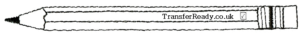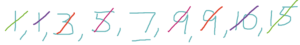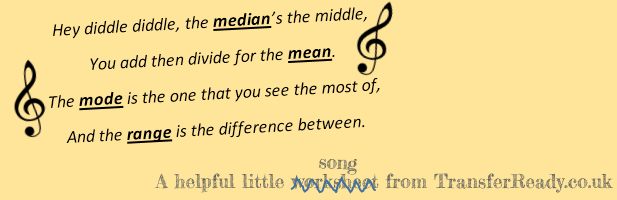###### Did you know that there are four different types of Average?

We consider there to be four types of average: mean, mode, median and range. Actually, range is a measure of spread or distribution but the others are our most common “measures of central tendency”.

##### Average means Mean

The mean is what most people think of when they hear the term ‘average’. It is found by adding up all of the numbers you have, and dividing the total by how many numbers are in the data set.##### Mode is Most Popular

The French word for fashion is mode. Do you remember the 1980’s electronic band, Depeche Mode? Enjoy the Silence? Well, their name was taken from a French Fashion magazine, which roughly translates as Fashion News or Fashion Update. Fashion = Popular. In conclusion, Mode means popular.

The type of average known as the mode is the most commonly occurring data point in the data set. The mode has some unique features;

• A data set can have more than one mode.
• A data set might not contain a mode.
• A mode might be qualitative, rather than quantitative – for instance, it could be a colour.
• The adjective form of mode is modal.##### Median is the middle

When you line up all the data points, in ascending order, the middle number is the median. This is much easier if there is an odd number of data points – in data sets with even numbers of data points, the median is the midpoint of the two middle numbers.

Generally, in these questions, begin by re-writing the list of numbers in ascending order (from smallest to largest) and then cross off the first and last numbers, working inwards in pairs until you get to the middle number.So, the median of these nine numbers is 7.Range is not really an average in the truest sense. It is actually a measure of how spread out a data set is. It is calculated by subtracting the smallest value from the biggest value.

##### So, in Summary…##### Questions: